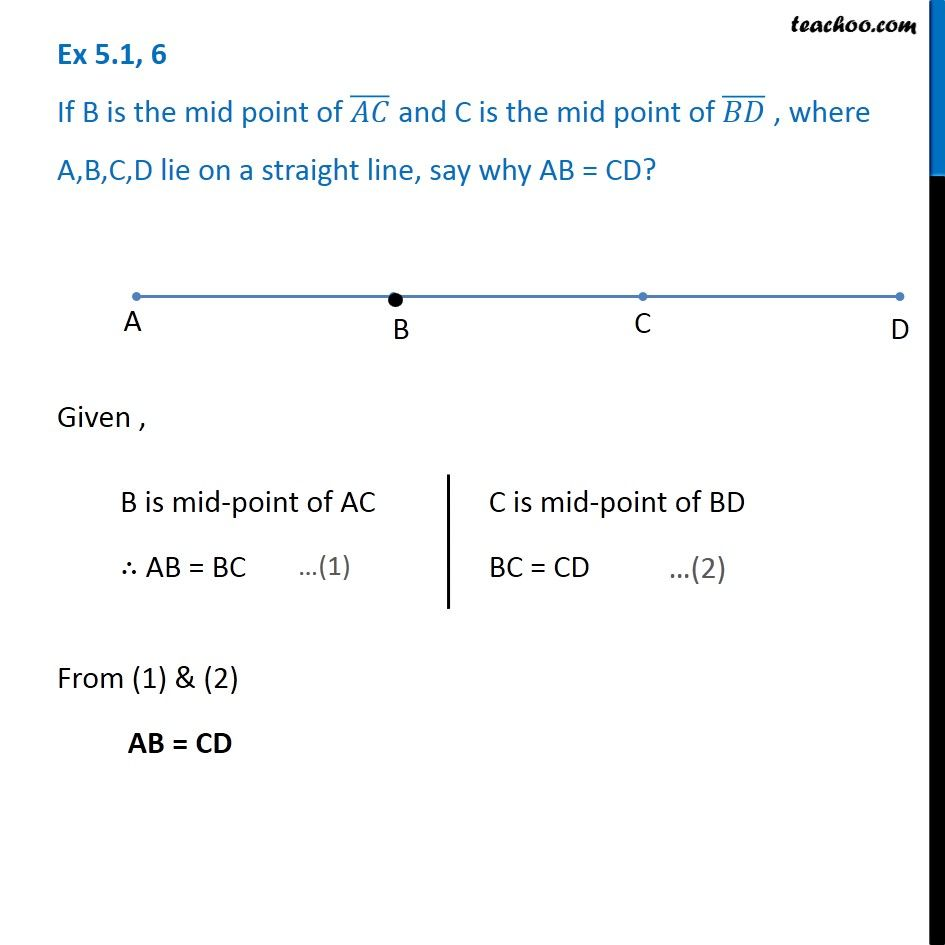1. Chapter 5 Class 6 Understanding Elementary Shapes
2. Concept wise
3. Measuring Line Segments

Transcript

Ex 5.1, 6 If B is the mid point of (𝐴𝐶) ̅ and C is the mid point of (𝐵𝐷) ̅ , where A,B,C,D lie on a straight line, say why AB = CD? Given , From (1) & (2) AB = CD B is mid-point of AC ∴ AB = BC C is mid-point of BD BC = CD

Measuring Line Segments